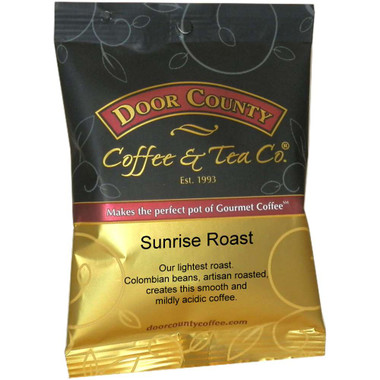•Quantity Decrease Quantity Increase Quantity Decrease Quantity
•Quantity Decrease Quantity Increase Quantity Decrease Quantity
\$49.95
\$54.95
•Quantity Decrease Quantity Increase Quantity Decrease Quantity
\$49.95
\$54.95
•Quantity Decrease Quantity Increase Quantity Decrease Quantity
\$49.95
\$54.95
•Quantity Decrease Quantity Increase Quantity Decrease Quantity
\$49.95
\$54.95
•Quantity Decrease Quantity Increase Quantity Decrease Quantity
\$49.95
\$54.95
•Quantity Decrease Quantity Increase Quantity Decrease Quantity
\$49.95
\$54.95
•Quantity Decrease Quantity Increase Quantity Decrease Quantity